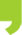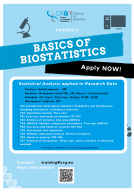## You are here

• You are here:
• Home > Events > Internal Training : Basics of Biostatistics 2023

# Internal Training : Basics of Biostatistics 2023

##Internal Training : Basics of Biostatistics 202321/04/2023 23/06/2023

## Internal Training : Basics of Biostatistics 2023

On-line

Description:

The course will give an overview of important concepts and methods used to analyse “Biomedical data”. The emphasis will be on the understanding of statistical concepts and their interpretation in a research framework. After a general introduction on probability theory and statistical inference, an emphasis will be made on common statistical methods. Particular cases will be used as illustrative examples.
The course will be composed of face-to-face theoretical lectures combined with practical sessions and additional short homework assignment.

Teacher: Hafid Laayouni (UPF)

Duration: 10 sessions (total 20h)

Schedule:  Each Friday from 21st April to 23rd June 2023, (10:00-12:00h, except first session that will be from 9:00-12:00h)

Time: 10:00-12:00am

Where: Zoom

Requirements: Participants need to have basic R programing skills.
If you don't have it, you can do this course starting 9th March:
Introduction to programming in R 2023
or  https://biocorecrg.github.io/CRG_RIntroduction_2021/

Application HERE

Application deadline: 1st of April 2023

AGENDA:

Introduction. Descriptive statistics. Probability and distributions. Sampling distribution. Confidence
intervals - Theory – 21stApril (9:00-12:00h)
Hypothesis testing. The t-test - Theory - 28th April
Exercises and Hands on sessions 1-2 - Practicum - 5th May
Analysis of variance. One-way ANOVA - Theory - 12th May
ANOVA. Multiple comparison procedures. Two-way ANOVA - Theory - 19th May
Hands on sessions 3-4 - Practicum - 26th May
Correlation and regression - Theory – 2nd June
Multiple regression analysis. Partial correlation - Theory - 9th June
Hands on sessions 7-8 - Practicum - 16th June
Effect Size and a summary of inference methods - Theory – 23rd June

At the end of the course, the students will be able to:

• Express a scientific question in a mathematical formulation possible to analyse with statistical method.
• To be confident about sampling methods and their impact on the quality of the data.
• Think on the basis of experimental design: replication, randomization and stratification.
• Think about the appropriate statistical method given the data we have (dependent and/or independent variable, continuous or categorical variables).
• Have a deep understanding of the meaning and interpretation of a test statistic and how to construct own statistic given data.
• Have a correct understanding of key words in basic statistic: null hypothesis, alternative hypothesis, error type I, error type II, statistical power, observational study, experimental study, causation and correlation, sampling error, random error, systematic error, sampling distribution, p-value, statistical and practical significance, effect size, statistical power.
• Correct interpretation of the result of a statistical test and been aware of the limitations of the applied methods.biostatistics_2023_2.pdf